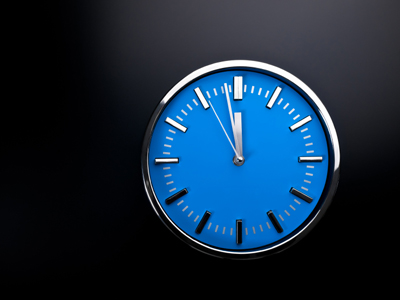Take time to play this quiz all about time!

# Time (Easy)

This 11-plus Maths quiz about time is going to look at the 24 hour clock. This clock is used extensively, for example: in computers, in the military and in transport.

Using the 24 hour clock removes all possibility of making a mistake with the time, for example: 'Meet me at 6 o'clock'. Is that 6 o'clock in the morning or in the evening? The use of 'am' and 'pm' doesn't always help because not all people know what they mean: pm = post meridiem = between 12 noon and 12 midnight; am = ante meridiem = between 12 midnight and 12 noon.

Some adults find the 24 hour clock difficult to remember. 16:18 is not as easy as nearly twenty past four, is it? It is a useful thing to learn and you should find it easier than adults. Why? Because you have grown up with computers which always show the time in 24 hours.

00:00 = 12:00 midnight (start of day)
01:00 = 1:00 a.m.
02:00 = 2:00 a.m.
03:00 = 3:00 a.m.
04:00 = 4:00 a.m.
05:00 = 5:00 a.m.
06:00 = 6:00 a.m.
07:00 = 7:00 a.m.
08:00 = 8:00 a.m.
09:00 = 9:00 a.m.
10:00 = 10:00 a.m.
11:00 = 11:00 a.m.
12:00 = 12:00 (noon)
13:00 = 1:00 p.m.
14:00 = 2:00 p.m.
15:00 = 3:00 p.m.
16:00 = 4:00 p.m.
17:00 = 5:00 p.m.
18:00 = 6:00 p.m.
19:00 = 7:00 p.m.
20:00 = 8:00 p.m.
21:00 = 9:00 p.m.
22:00 = 10:00 p.m.
23:00 = 11:00 p.m.
00:00 = 12:00 midnight (end of day)
1.
Using the 24-hour conversion table, how would the time appear on the 24 hour clock?
6:30 am
18:30
06:30
6:30
8:30
This is read as 'zero six thirty hours'. TIP: Between midnight and noon, you don't have to do any conversion, e.g. 5:40 am = 05:40
2.
Using the 24-hour conversion table, how would the time appear on the 24 hour clock?
7:00 pm
19:00
07:00
7:00
19
This is read as 'nineteen hundred hours'. TIP: After 12 noon until midnight, you add 12 to the hour, e.g. 5:40 pm = 17:40
3.
Using the 24-hour conversion table, how would the time appear on the 24 hour clock?
6:35 am
16:35
6:35
18:35
06:35
This is read as 'zero six thirty-five hours'
4.
Using the 24-hour conversion table, how would the time appear on the 24 hour clock?
9:00 pm
9:00
21
09:00
21:00
This is read as 'twenty-one hundred hours'
5.
Using the 24-hour conversion table, how would the time appear on the 24 hour clock?
11:30 am
11:30
23:30
011:30
22:30
This is read as 'eleven thirty hours'
6.
Using the 24-hour conversion table, how would the time appear on the 24 hour clock?
5:15 pm
17:15
05:15
5:15
07:15
This is read as 'seventeen fifteen hours'
7.
Using the 24-hour conversion table, how would the time appear on the 24 hour clock?
4 pm
04
04:00
16:00
16
This is read as 'sixteen hundred hours'
8.
Using the 24-hour conversion table, how would the time appear on the 24 hour clock?
10:45 pm
22:45
10:45
010:45
23:45
This is read as 'twenty-two forty-five hours'
9.
Using the 24-hour conversion table, how would the time appear on the 24 hour clock?
3:15 am
15:15
03:15
3:15
003:15
This is read as 'zero three hundred fifteen hours'
10.
Using the 24-hour conversion table, how would the time appear on the 24 hour clock?
12:00 midnight
12
0:00
0:0
00:00
This is read as 'zero zero hundred hours'. Note: The day begins at midnight, and the last minute of the day begins at 23:59 and ends at 24:00 BUT this is shown as 00:00. Some people do use 24:00, for example: 'Open from 00:00 to 24:00' - this shows that the business is open for the whole 24 hour period. To use 'Open from 00:00 to 00:00' might be a bit confusing. In general, avoid using 24:00 when telling the time
Author:  Frank Evans